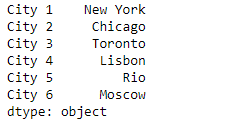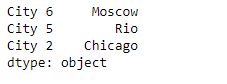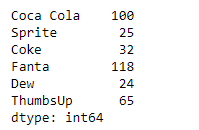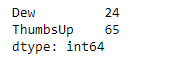Related Articles

# Python | Pandas Series.sample()

• Last Updated : 07 Feb, 2019

Pandas series is a One-dimensional ndarray with axis labels. The labels need not be unique but must be a hashable type. The object supports both integer- and label-based indexing and provides a host of methods for performing operations involving the index.

Pandas` Series.sample()` function return a random sample of items from an axis of object. We can also use random_state for reproducibility.

Syntax: Series.sample(n=None, frac=None, replace=False, weights=None, random_state=None, axis=None)

Parameter :
n : Number of items from axis to return.
frac : Fraction of axis items to return.
replace : Sample with or without replacement.
weights : Default ‘None’ results in equal probability weighting.
random_state : Seed for the random number generator (if int), or numpy RandomState object.
axis : Axis to sample.

Returns : Series or DataFrame

Example #1: Use `Series.sample()` function to draw random sample of the values from the given Series object.

 `# importing pandas as pd``import` `pandas as pd`` ` `# Creating the Series``sr ``=` `pd.Series([``'New York'``, ``'Chicago'``, ``'Toronto'``, ``'Lisbon'``, ``'Rio'``, ``'Moscow'``])`` ` `# Create the Datetime Index``index_ ``=` `[``'City 1'``, ``'City 2'``, ``'City 3'``, ``'City 4'``, ``'City 5'``, ``'City 6'``]`` ` `# set the index``sr.index ``=` `index_`` ` `# Print the series``print``(sr)`

Output :Now we will use `Series.sample()` function to draw a random sample of values from the given Series object.

 `# Draw random sample of 3 values``selected_cities ``=` `sr.sample(n ``=` `3``)`` ` `# Print the returned Series object``print``(selected_cities)`

Output :As we can see in the output, the `Series.sample()` function has successfully returned a random sample of 3 values from the given Series object.

Example #2: Use `Series.sample()` function to draw random sample of the values from the given Series object.

 `# importing pandas as pd``import` `pandas as pd`` ` `# Creating the Series``sr ``=` `pd.Series([``100``, ``25``, ``32``, ``118``, ``24``, ``65``])`` ` `# Create the Index``index_ ``=` `[``'Coca Cola'``, ``'Sprite'``, ``'Coke'``, ``'Fanta'``, ``'Dew'``, ``'ThumbsUp'``]`` ` `# set the index``sr.index ``=` `index_`` ` `# Print the series``print``(sr)`

Output :Now we will use `Series.sample()` function to select a random sample of size equivalent to 25% of the size of the given Series object.

 `# Draw random sample of size of 25 % of the original object``selected_items ``=` `sr.sample(frac ``=` `0.25``)`` ` `# Print the returned Series object``print``(selected_items)`

Output :As we can see in the output, the `Series.sample()` function has successfully returned a random sample of 2 values from the given Series object, which is 25% of the size of the original series object.

Attention geek! Strengthen your foundations with the Python Programming Foundation Course and learn the basics.

To begin with, your interview preparations Enhance your Data Structures concepts with the Python DS Course. And to begin with your Machine Learning Journey, join the Machine Learning – Basic Level Course

My Personal Notes arrow_drop_up